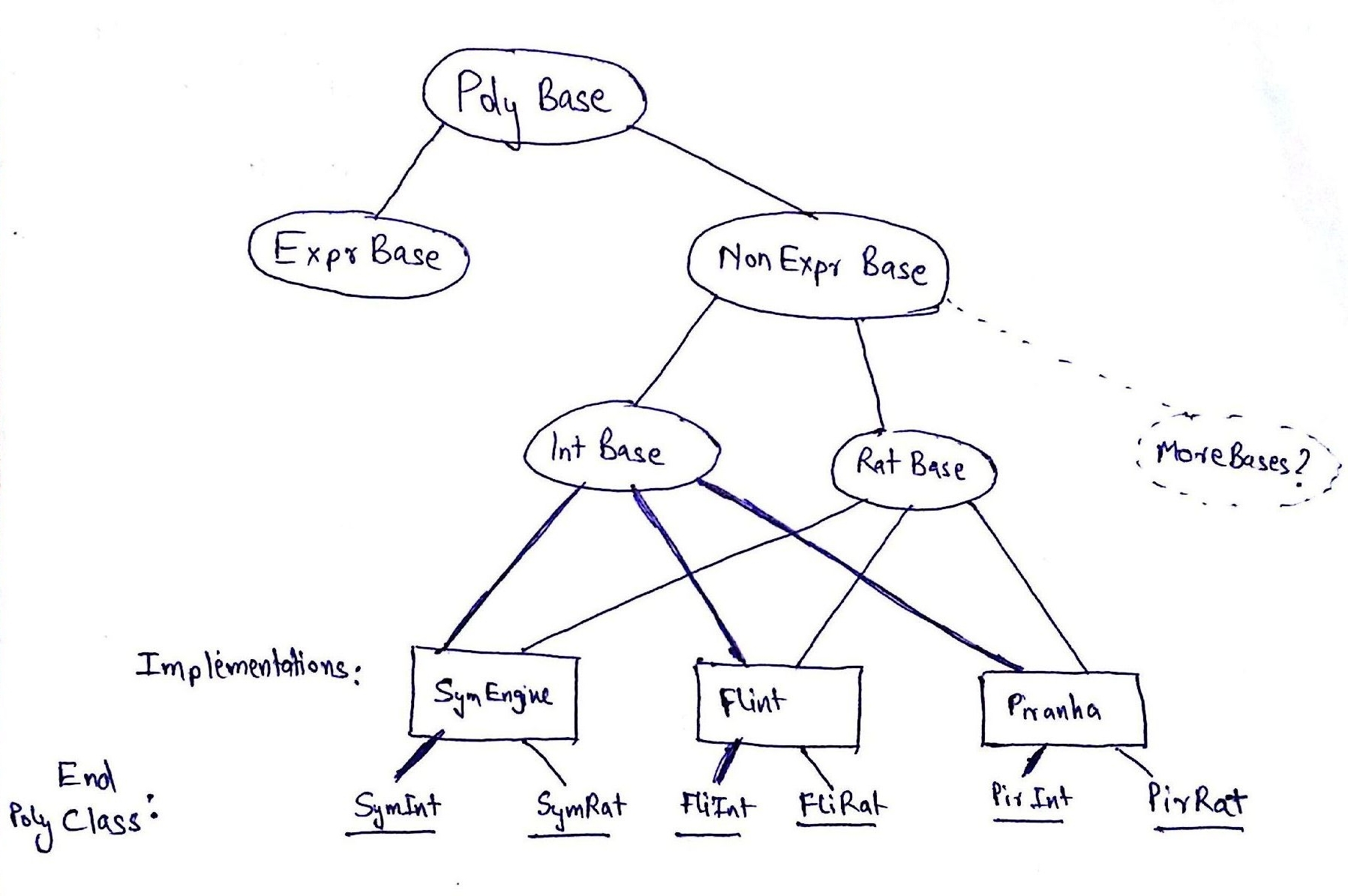# Rational Polynomials

### Overview

This last week was divided into two parts. The first half was spent in fixing miscellaneous issue with the last PR, regarding the basic to polynomial converisons. The second half was spent in thinking of how SymEngine should store rational polynomials (polynomials with rational coefficients) and how infact should they be implemented.

### Leftovers from Basic Conversions

As mentioned, the first half of my week was spent finalizing the basic to polynomial conversions PR.

• Using `divnum` and `mulnum` methods instead of using `rcp_cast<Number>` on `mul` and `div` respectively. These made the code much more readable too.

• Use `static_cast<const Rational &>(*rat_rcp).get_den()` instead of `rcp_static_cast<const Rational>(rat_rcp)->get_den()`. The logic behind this is, that in the second case, another `RCP` is being constructed, and the `get_den` function is called on this new RCP. This is unecessary and can be avoided using the former approach.

• At many places within the visitor pattern, I was calling the external function `basic_to_poly`. This was not wrong, but caused necessary overhead and recursion, which is not usually desired. Isuru suggested that I can reuse the visitor and successfully avoid the recursion. Here’s an example

``````dict_type _basic_to_poly(basic, gen)
{
BasicToPolyV v;
v.apply(basic, gen);
}

// dict is the answer we will be returning
{
for (auto const &it : x.dict_)
dict += _basic_to_upoly(it, gen);
}
``````

becomes this

``````// dict is the answer we will be returning
{
dict_type res;
for (auto const &it : x.dict_)
res += BasicToPolyV::apply(it, gen);
dict = std::move(res);
}
``````
• There was also a discussion on how the visitor for `Pow` in `find_gen_pow` was implemented. Isuru pointed out, how it would give wrong answers in the cases he put forward. But I had thought of other cases (like `2**(2**(x+1))`) which would would then not work, if the code was changed. These were special type of cases where the expression could be ‘simplified’. There’s some discussion on the issue here.

### Starting Rational Polynomials

I began thinking of how the polynomials with rational coefficients class should be implemented. In Flint, the `fmpq_poly` class is implemented as a `fmpz_poly` class with another just another integer acting as the common denominatior. This kind of implementation saves up on a lot of time! (think of canonicalization of coefficients, after multiplication) But this kind of implementation would require a lot of extra code be written, and we could not reuse any of the code we have already written. So, after discussion with Isuru, he suggested that I can go ahead with the trivial implementation of using a map from `uint` to `rational_class`.

``````class URatDict : ODictWrapper<uint, rational_class>
``````

Add in some constructors and that’s it! The internal container for rational polynomials in SymEngine is ready, because of the way we had strcutured the earlier code.

Now the question was how the end classes should be implemented (i.e. `URatPoly`) If you think about it, the methods of both the rational and integer polynomials will look exactly the same. `from_vec`, `container_from_dict`, `eval`, `compare` will all look the same with minor differences. The minor differences are only what type of container is being used (`URatDict` or `UIntDict`?) and what coefficient class is being used (`integer_class` or `rational_class`?) Infact, I checked this for our other polynomial types Flint and Piranha and it holds true over there too!

So, what came to mind was that all the methods had to be reused! Thus I formed a `USymEnginePoly` class which acts as the ‘SymEngine’ implementation of polynomials. You can pass it a `BaseType` from which it will deduce what type of polynomial would be constructed. Ideally, all you need to do now for implenting end polynomial classes is choose a implementation type (SymEngine or Piranha or Flint?) and a polynomial type (Integer or Rational?)

Here’s some pseudo code for the class structure aimed at

``````// the base
template <typename Container>
class UPolyBase : Basic

// super class for all non-expr polys, all methods which are
// common for all non-expr polys go here eg. degree, eval etc.
template <typename Cont, typename C>
class UNonExprPoly : UPolyBase<Cont>

// the specialized non-expr classes
template <typename D>
class UIntPolyBase : UNonExprPoly<D, integer_class>
// and
template <typename D>
class URatPolyBase : UNonExprPoly<D, rational_class>

// a specific implementation
// similar classes like UPiranhaPoly/UFlintPoly
template <template <typename X> class BaseType, typename D>
class USymEnginePoly : BaseType<D>

// end classes (few examples)
class UIntPoly : USymEnginePoly<UIntDict, UIntPolyBase>
class URatPoly : USymEnginePoly<URatDict, URatPolyBase>
class UIntPolyPir : UPiranhaPoly<piranha::poly, UIntPolyBase>
class URatPolyFlint : UFlintPoly<flint::poly, URatPolyBase>
``````

Here’s a bonus picture!I hope to get all the rational polynomials ready and tested in the next two to three days. The existing work can be seen in #1028

Tata!